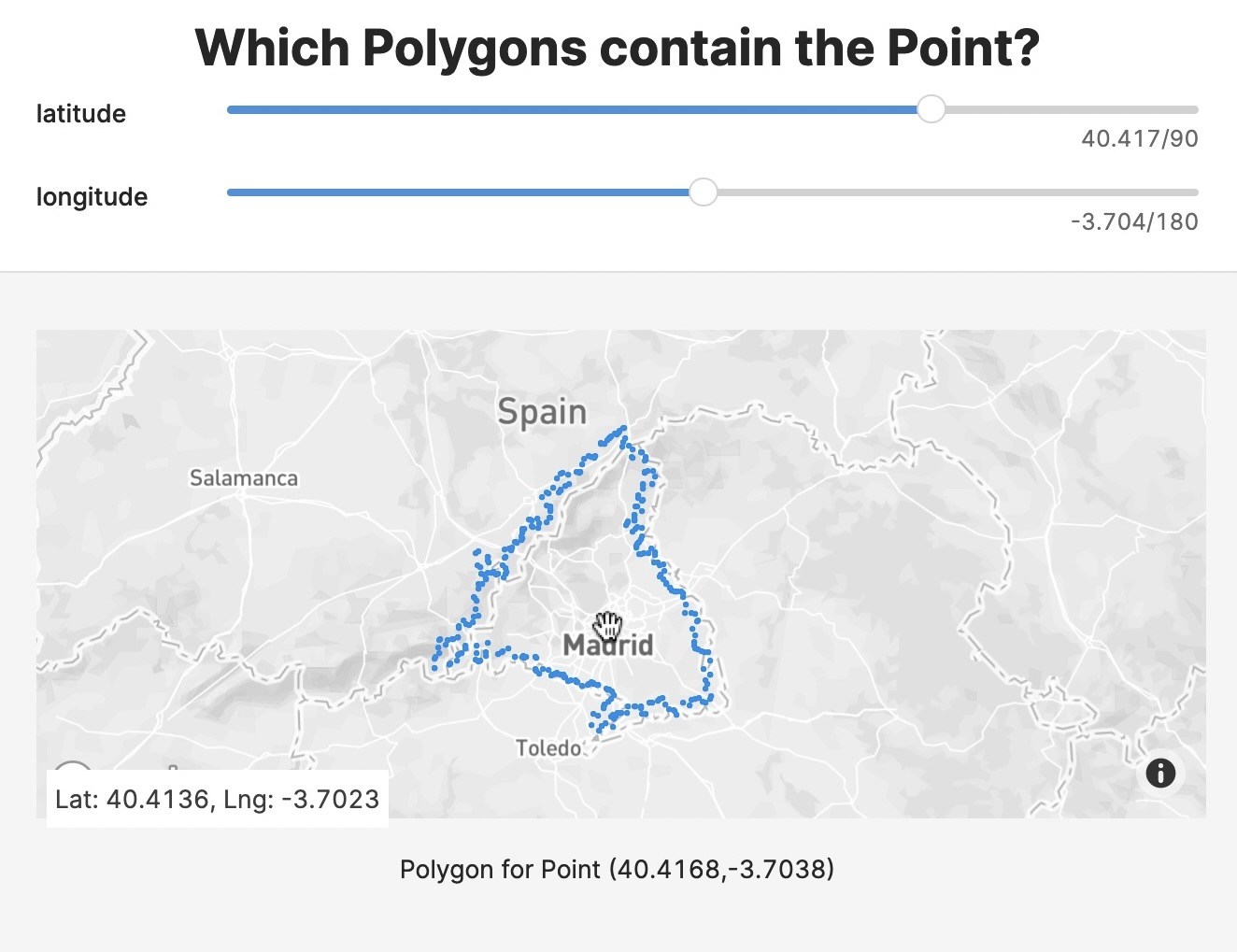🚀
Introducing Versions: Develop data products using Git. Join the waitlist

# Spatial Indexing aids Finding which Polygons contain a Point

Speed up your queries by using a spatial index to select fewer polygons before testing if a point is inside a polygon.Finding the polygons that contain a given point is a common geospatial operation but can be complex. Speed up finding polygons using the geohash trick. Geohash subdivides the Earth’s surface into buckets of grid shape and encodes each cell into a short string of letters and digits. It is a hierarchical data structure, so the longer the geohash string, the more precise the geographic location.

## Code to List Polygons that Contain a Point

We use ClickHouse’s functions for working with geohash:

• {% code-line %}geohashEncode(longitude, latitude, [precision]){% code-line-end %}
• {% code-line %}geohashesInBox(longitude_min, latitude_min, longitude_max, latitude_max, precision){% code-line-end %}

The schema of the data used here is an integer id and a multipolygon as an array of single polygons, each an array of tuples.

First we generate the materialized view {% code-line %}geo_index{% code-line-end %} with a mapping {% code-line %}geohash{% code-line-end %} -> {% code-line %}Array(geoname_id){% code-line-end %}. That is, for each geohash there is an array of ids whose polygon(s) are partly in the geohash polygon.

In order to calculate all the {% code-line %}geohash{% code-line-end %} covered by a polygon, the polygon bounding box is calculated (longitude is wrapped to [-180, 180]) and the box is used with {% code-line %}geohashesInBox{% code-line-end %} to return a list of polygons.

Geohash level is decided based on the polygon area. In this example, polygons are pretty large, so a low number (3) is used. For small polygons, a higher level of detail should be used.

When querying data the geohash of the point is calculated from the {% code-line %}geo_index{% code-line-end %} table, the list of {% code-line %}geoname_ids{% code-line-end %} is retrieved and this list of candidates is used to get the actual polygons.

Note that the candidates list is not the final one, the point can have the same {% code-line %}geohash{% code-line-end %} but be outside of the polygon, so we need to check if the point is inside the polygon using {% code-line %}pointInPolygon(){% code-line-end %}.

The steps are:

• calculate the geohash of the current point. The geohash level should match the one used in {% code-line %}geo_index_mv{% code-line %} (here 3).
• calculate the candidate polygons based on the geohash index table
• check the candidate polygons one by one with {% code-line %}pointInPolygon{% code-line-end %}, since geohash is a lossy filter. This function is pretty expensive but, thanks to the index, you only query the relevant part of the data.

## Experimental Geo Types

ClickHouse now has experimental_geo_types:

• Point: (x,y) - a tuple of Float64s
• Ring: a simple polygon without holes - an array of points
• Polygon: a polygon with holes - an array of rings. The first element of the outer array is the outer shape of the polygon and all the following elements are holes.
• MultiPolygon: multiple polygons - an array of polygons.

These will open the door to even faster queries in the future.

## Speed Up with Spatial Indexing

By using a spatial indexing you test far fewer polygons and so speed up your query. In the same way that indexing your Data Sources with sorting keys improves query speed, spatial indexing also increases efficiency.

If you have an interesting use case, write to us at hi@tinybird.co, sign up to Tinybird and easily build your own data apps. If you already have an account, join our community Slack. Looking forward to seeing you there.